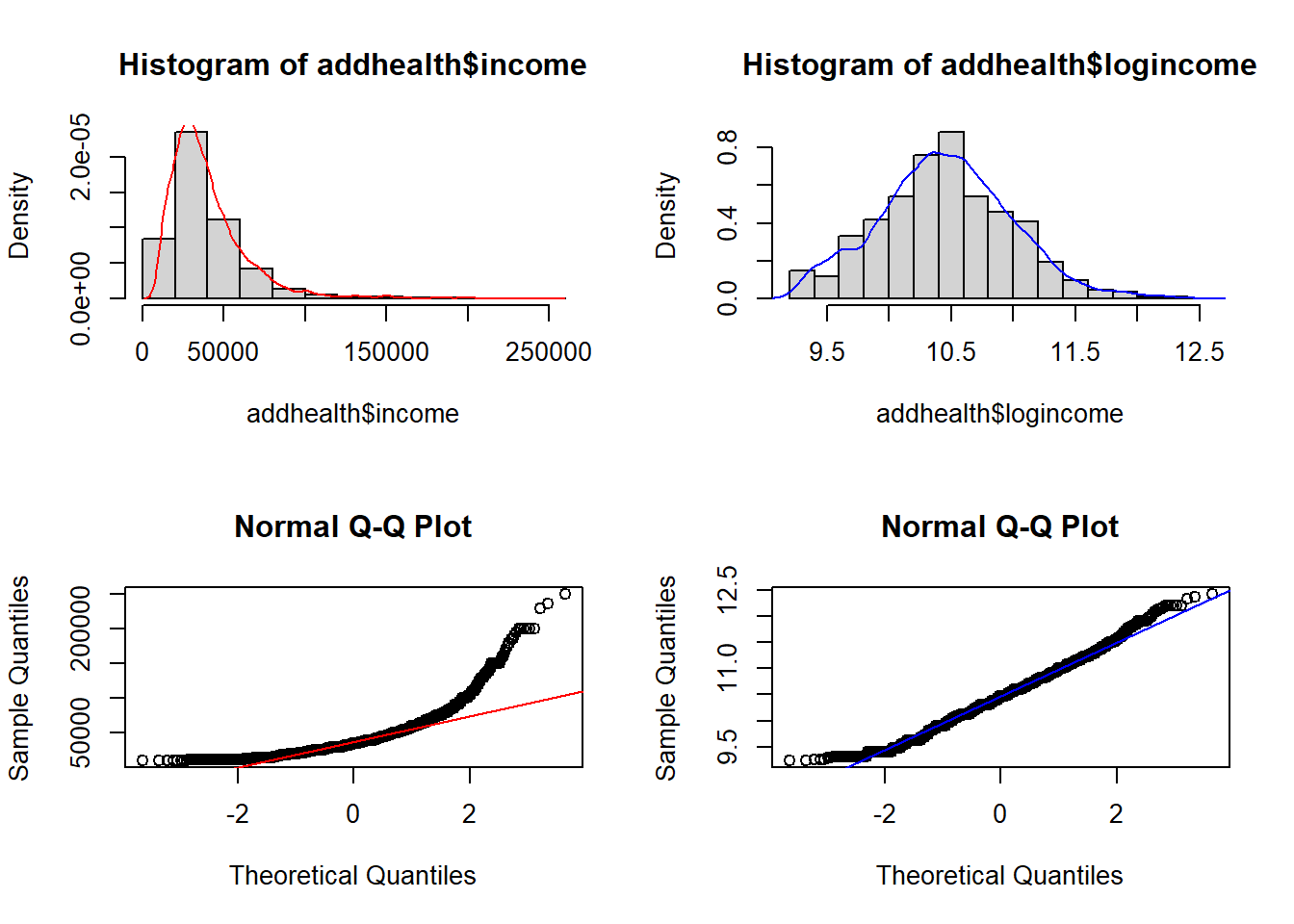## 10.4 Log-linear models

A log-linear model is when the log of the response variable is modeled using a linear combination of predictors.

$ln(Y) \sim XB +\epsilon$

Recall that in statistics, when we refer to the log, we mean the natural log ln.

This type of model is often use for Poisson models also (Section 10.5.0.1).

Why are we transforming the outcome? Typically to achieve normality when the response variable is highly skewed.

Interpreting results

This is hands down the best reference that describes how to interpret the results when your response, predictor, or both variables are log transformed.

https://stats.idre.ucla.edu/other/mult-pkg/faq/general/faqhow-do-i-interpret-a-regression-model-when-some-variables-are-log-transformed/

### 10.4.1 Example

We are going to analyze personal income from the AddHealth data set. First I need to clean up, and log transform the variable for personal earnings H4EC2 by following the steps below in order.

1. Remove values above 999995 (structural missing).
2. Create a new variable called income, that sets all values of personal income to be NA if below the federal poverty line.
• First set income= H4EC2
• Then set income to missing, if H4EC2 < 10210 (the federal poverty limit from 2008)
3. Then create a new variable: logincome that is the natural log (ln) of income. e.g. addhealth$logincome = log(addhealth$income)

Why are we transforming income? To achieve normality.

par(mfrow=c(2,2))
hist(addhealth$income, probability = TRUE); lines(density(addhealth$income, na.rm=TRUE), col="red")
hist(addhealth$logincome, probability = TRUE); lines(density(addhealth$logincome, na.rm=TRUE), col="blue")
qqnorm(addhealth$income); qqline(addhealth$income, col="red")
qqnorm(addhealth$logincome); qqline(addhealth$logincome, col="blue")Identify variables

• Quantitative outcome that has been log transformed: Income (variable logincome)
• Binary predictor: Ever smoked a cigarette (variable eversmoke_c)
• Binary confounder: Gender (variable female_c)

The mathematical multivariable model looks like:

$ln(Y) \sim \beta_{0} + \beta_{1}x_{1} + \beta_{2}x_{2}$

Similar to logistic regression, we need to exponentiate the regression coefficient before we can interpret the number as a percentage change in $$Y$$ for a unit increase in $$x_{j}$$.

• $$b_{j}<1$$ : The expected value of $$Y$$ for when $$x=0$$ is $$1 - e^{b_{j}}$$ percent lower than when $$x=1$$
• $$b_{j} \geq 1$$ : The expected value of $$Y$$ for when $$x=0$$ is $$e^{b_{j}}$$ percent higher than when $$x=1$$
ln.mod.2 <- lm(logincome~wakeup + female_c, data=addhealth)
summary(ln.mod.2) %>% pander()
Estimate Std. Error t value Pr(>|t|)
(Intercept) 10.65 0.026 409.8 0
wakeup -0.01491 0.003218 -4.633 3.73e-06
female_cFemale -0.1927 0.017 -11.34 2.564e-29
Fitting linear model: logincome ~ wakeup + female_c
Observations Residual Std. Error $$R^2$$ Adjusted $$R^2$$
3813 0.5233 0.03611 0.0356
1-exp(confint(ln.mod.2)[-1,])
##                     2.5 %      97.5 %
## wakeup         0.02099299 0.008561652
## female_cFemale 0.20231394 0.147326777
• For every hour later one wakes up in the morning, one can expect to earn 1-exp(-0.015) = 1.4% less income than someone who wakes up one hour earlier. This is after controlling for gender.
• Females have on average 1-exp(-0.19) = 17% percent lower income than males, after controlling for the wake up time.

Both gender and time one wakes up are significantly associated with the amount of personal earnings one makes. Waking up later in the morning is associated with 1.4% (95% CI 0.8%-2%, p<.0001) percent lower income than someone who wakes up one hour earlier. Females have 17% (95% CI 15%-20%, p<.0001) percent lower income than males.